# How To Concatenate Cells If Same Value Exists in Another Column in Excel

This post will guide you how to concatenate cells if same value in another column using an VBA macro in Excel. How do I merge cells if same value exists in another column using formula and filter feature in Excel 2013/2016.

Assuming that you have a list of data in range A1:B6, you wish to concatenate cells in column B if the approatiate cell values are same in the column A. How to do it. And you can use an VBA Macro to quickly concatenate cells based on your conditaion. or you can use another method based on Formulas and filter function.

### Concatenate Cells If Same value Exists in Another Column using Formulas

To concatenate cells in range B1:B6 based on the cell values in range A1:A6 if they are same, you can use two formulas based on the `IF function` and the `Concatenate function`. Just do the following steps:

#1 select one blank cell (Cell c2) beside range B1:B6, and then type the following formula, press `Enter` key on your keyboard to apply it.

`=IF(A2<>A1,B2,C1 & "," & B2)`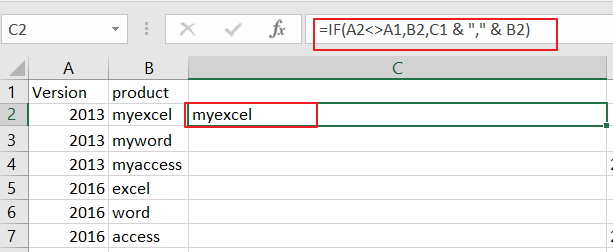#2 drag the AutoFill Handle in cell C2 down to other cells to apply this formula.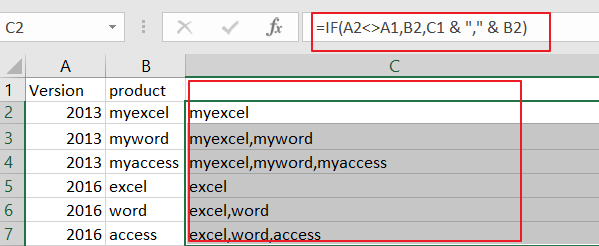#3 you need to type anothe formula in Cell D2, then press `Enter` key.

`=IF(A2<>A3,CONCATENATE(A2,",",C2),"")`

#4 drag the AutoFill Handle in cell D2 down to other cells to apply this formula. You would see that the cell values in range B1:B6 have been concatenated in range D1:D6.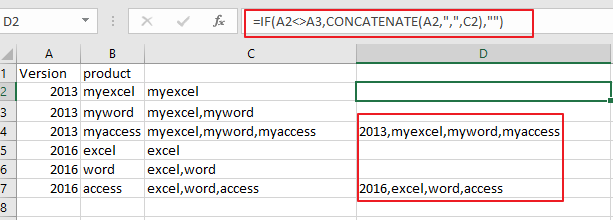#5 Now you need to filter out all blank cells in range D1:D6. And select all cell in Range D1:D6, and go to `Data` tab, click `Filter` button under` Sort&Filter` group. and a Filter array icon will be added in the first cell in range D1:D6.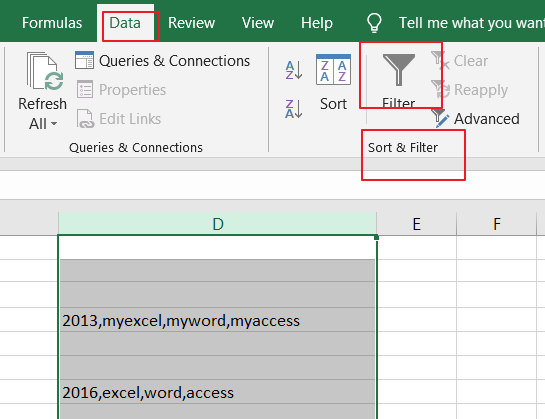#6 click the Filter icon in Cell D1, and uncheck `Blanks` checkbox, and then click `Ok` button.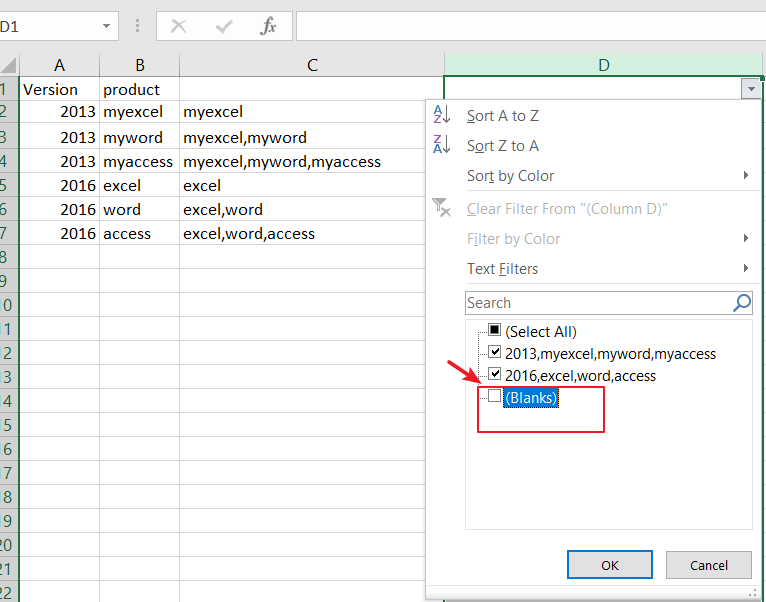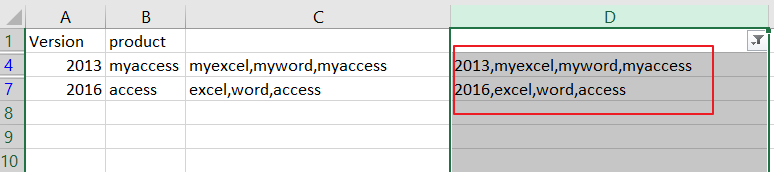### Concatenate Cells If Same Value Exists in Another Column using VBA

You can also use an Excel VBA Macro to concatenate cells in Range B1:B6 if same values exists in range A1:A6. Just do the following steps:

Step1: open your excel workbook and then click on “`Visual Basic`” command under `DEVELOPER` Tab, or just press “`ALT+F11`” shortcut.Step2: then the “`Visual Basic Editor`” window will appear.

Step3: click “`Insert`” ->”`Module`” to create a new module.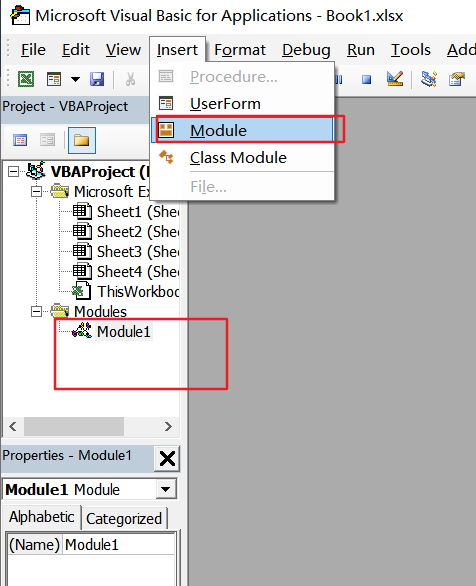Step4: paste the below VBA code  into the code window. Then clicking “`Save`” button.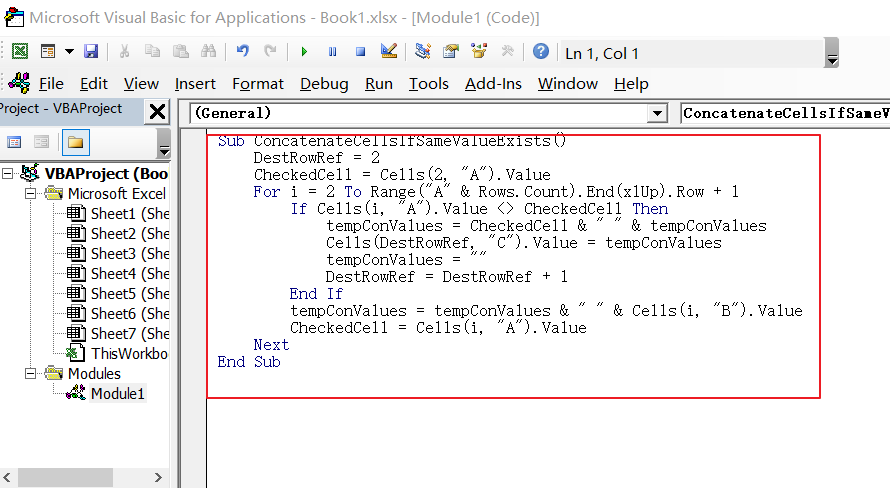```Sub ConcatenateCellsIfSameValueExists()
DestRowRef = 2
CheckedCell = Cells(2, "A").Value
For i = 2 To Range("A" & Rows.Count).End(xlUp).Row + 1
If Cells(i, "A").Value <> CheckedCell Then
tempConValues = CheckedCell & " " & tempConValues
Cells(DestRowRef, "C").Value = tempConValues
tempConValues = ""
DestRowRef = DestRowRef + 1
End If
tempConValues = tempConValues & " " & Cells(i, "B").Value
CheckedCell = Cells(i, "A").Value
Next
End Sub```

Note: this VBA Macro will compare cell values in column A, and then concatenate cell values in Column B, and palce the concatenated values into column C, and started from Cell C2.

Step5: back to the current worksheet, click on `Macros` button under `Code` group. then click Run button.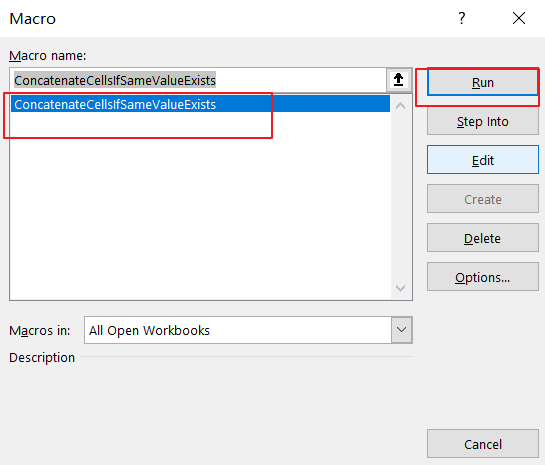Step6: let’s see the last result: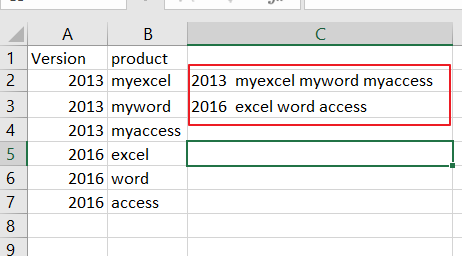### Related Functions

• Excel IF function
The Excel IF function perform a logical test to return one value if the condition is TRUE and return another value if the condition is FALSE. The IF function is a build-in function in Microsoft Excel and it is categorized as a Logical Function.The syntax of the IF function is as below:= IF (condition, [true_value], [false_value])….
Related Posts

Find and Replace Multiple Values

This post will guide you how to find and replace multiple values at once with VBA macro or using formula  in Excel. How do I make multiple find and replace in Excel. Suppose that you have a few cells containing ...

VBA Macro For VLOOKUP From Another Sheet

In the previous post, you should know that how to fix or remove the #N/A error when using VLOOKUP formula to lookup value from another sheet. And this post will show you how to use VBA code to vlookup data ...

How To Insert Comments in Protected Worksheet in Excel

This post will show you how to allow comments in a protected worksheet in Excel. You can easily to insert comments into cells in a normal worksheet in Excel, but if want to insert a comment in a worksheet that ...

How To Convert Text to Upper Cases(Using VBA) in Excel

This post will show you how to switch from lower case to upper case in Excel. and I am going to show you two different ways of converting text to upper cases using formula or VBA macro in Excel 2013,Excel ...

How To Hide Every Other Row in Excel (Using VBA)

This post will show you how to hide alternate rows or columns in Excel or how to hide every third, fourth, fifth row or column in Excel. If you want to hide every other row in your current worksheet, how ...

How to Disable the Save As Prompt in Excel

This post will show you how to use a VBA Macro to save an Excel file and overwrite any existing file without a prompt so that you are going to get the little window that says file already exists do ...

How to Count Cells that Contain even or odd numbers in Excel

This post will guide you how to count the number of cells that contain odd or even numbers within a range of cells using a formula in Excel 2013/2016.How do I count cells that contain odd numbers through the use ...

How to Count Cells that Contain negative Numbers in Excel

This post will guide you how to count the number of cells that contain negative numbers within a range of cells using a formula in Excel 2013/2016.You can count the number of negative numbers in your data using easy functions ...

How to Count Cells Are Not Blank or Empty in Excel

This post will guide you how to count cells that are not blank or empty in a given range cells using a formula in Excel 2013/2016.How do I count the number of cells that are not blank in a particular ...

How to Count Cells Less Than a Specific Value in Excel

This post will guide you how to count the number of cells less than a particular numeric value in a given range cells using a formula in Excel 2013/2016. How do I count cells that are less than a specific ...

Sidebar